/scikit-learn

# sklearn.cluster.AgglomerativeClustering

`class sklearn.cluster.AgglomerativeClustering(n_clusters=2, affinity=’euclidean’, memory=None, connectivity=None, compute_full_tree=’auto’, linkage=’ward’, pooling_func=’deprecated’)` [source]

Agglomerative Clustering

Recursively merges the pair of clusters that minimally increases a given linkage distance.

Read more in the User Guide.

Parameters: `n_clusters : int, default=2` The number of clusters to find. `affinity : string or callable, default: “euclidean”` Metric used to compute the linkage. Can be “euclidean”, “l1”, “l2”, “manhattan”, “cosine”, or ‘precomputed’. If linkage is “ward”, only “euclidean” is accepted. `memory : None, str or object with the joblib.Memory interface, optional` Used to cache the output of the computation of the tree. By default, no caching is done. If a string is given, it is the path to the caching directory. `connectivity : array-like or callable, optional` Connectivity matrix. Defines for each sample the neighboring samples following a given structure of the data. This can be a connectivity matrix itself or a callable that transforms the data into a connectivity matrix, such as derived from kneighbors_graph. Default is None, i.e, the hierarchical clustering algorithm is unstructured. `compute_full_tree : bool or ‘auto’ (optional)` Stop early the construction of the tree at n_clusters. This is useful to decrease computation time if the number of clusters is not small compared to the number of samples. This option is useful only when specifying a connectivity matrix. Note also that when varying the number of clusters and using caching, it may be advantageous to compute the full tree. `linkage : {“ward”, “complete”, “average”, “single”}, optional (default=”ward”)` Which linkage criterion to use. The linkage criterion determines which distance to use between sets of observation. The algorithm will merge the pairs of cluster that minimize this criterion. ward minimizes the variance of the clusters being merged. average uses the average of the distances of each observation of the two sets. complete or maximum linkage uses the maximum distances between all observations of the two sets. single uses the minimum of the distances between all observations of the two sets. `pooling_func : callable, default=’deprecated’` Ignored. Deprecated since version 0.20: `pooling_func` has been deprecated in 0.20 and will be removed in 0.22. `labels_ : array [n_samples]` cluster labels for each point `n_leaves_ : int` Number of leaves in the hierarchical tree. `n_components_ : int` The estimated number of connected components in the graph. `children_ : array-like, shape (n_samples-1, 2)` The children of each non-leaf node. Values less than `n_samples` correspond to leaves of the tree which are the original samples. A node `i` greater than or equal to `n_samples` is a non-leaf node and has children `children_[i - n_samples]`. Alternatively at the i-th iteration, children[i] and children[i] are merged to form node `n_samples + i`

#### Examples

```>>> from sklearn.cluster import AgglomerativeClustering
>>> import numpy as np
>>> X = np.array([[1, 2], [1, 4], [1, 0],
...               [4, 2], [4, 4], [4, 0]])
>>> clustering = AgglomerativeClustering().fit(X)
>>> clustering
AgglomerativeClustering(affinity='euclidean', compute_full_tree='auto',
pooling_func='deprecated')
>>> clustering.labels_
array([1, 1, 1, 0, 0, 0])
```

#### Methods

 `fit`(X[, y]) Fit the hierarchical clustering on the data `fit_predict`(X[, y]) Performs clustering on X and returns cluster labels. `get_params`([deep]) Get parameters for this estimator. `set_params`(**params) Set the parameters of this estimator.
`__init__(n_clusters=2, affinity=’euclidean’, memory=None, connectivity=None, compute_full_tree=’auto’, linkage=’ward’, pooling_func=’deprecated’)` [source]
`fit(X, y=None)` [source]

Fit the hierarchical clustering on the data

Parameters: `X : array-like, shape = [n_samples, n_features]` Training data. Shape [n_samples, n_features], or [n_samples, n_samples] if affinity==’precomputed’. `y : Ignored` self
`fit_predict(X, y=None)` [source]

Performs clustering on X and returns cluster labels.

Parameters: `X : ndarray, shape (n_samples, n_features)` Input data. `y : Ignored` not used, present for API consistency by convention. `labels : ndarray, shape (n_samples,)` cluster labels
`get_params(deep=True)` [source]

Get parameters for this estimator.

Parameters: `deep : boolean, optional` If True, will return the parameters for this estimator and contained subobjects that are estimators. `params : mapping of string to any` Parameter names mapped to their values.
`set_params(**params)` [source]

Set the parameters of this estimator.

The method works on simple estimators as well as on nested objects (such as pipelines). The latter have parameters of the form `<component>__<parameter>` so that it’s possible to update each component of a nested object.

Returns: self

## Examples using `sklearn.cluster.AgglomerativeClustering`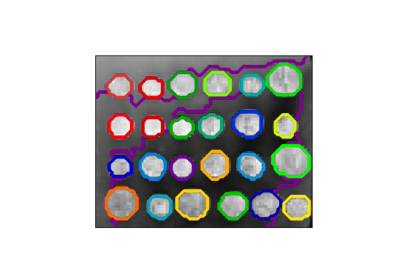A demo of structured Ward hierarchical clustering on an image of coins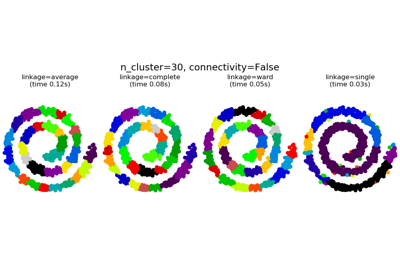Agglomerative clustering with and without structure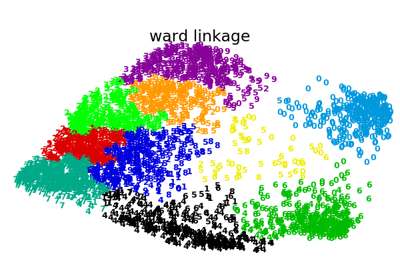Various Agglomerative Clustering on a 2D embedding of digits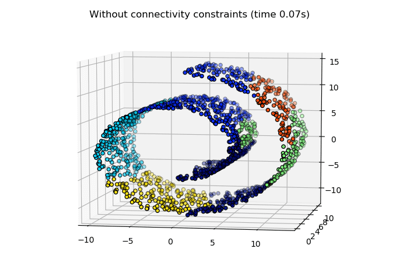Hierarchical clustering: structured vs unstructured ward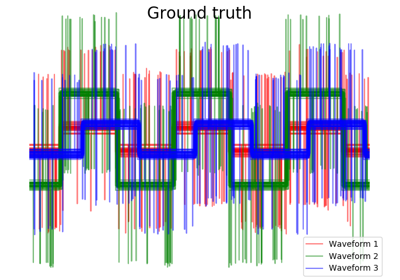Agglomerative clustering with different metrics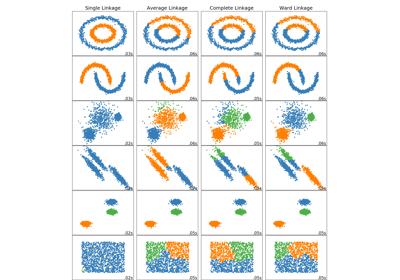Comparing different hierarchical linkage methods on toy datasets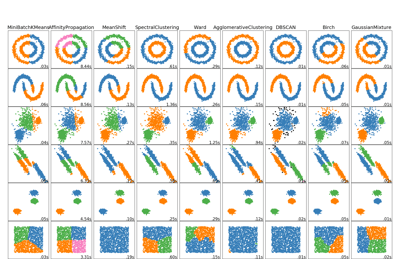Comparing different clustering algorithms on toy datasets